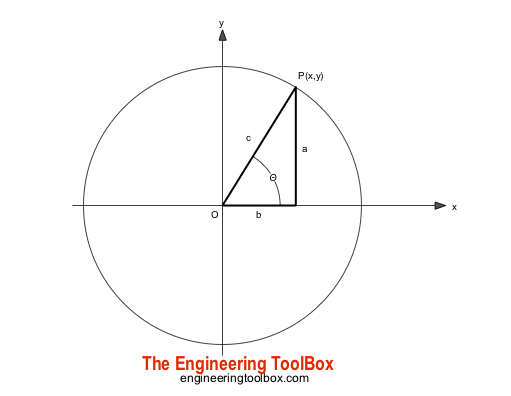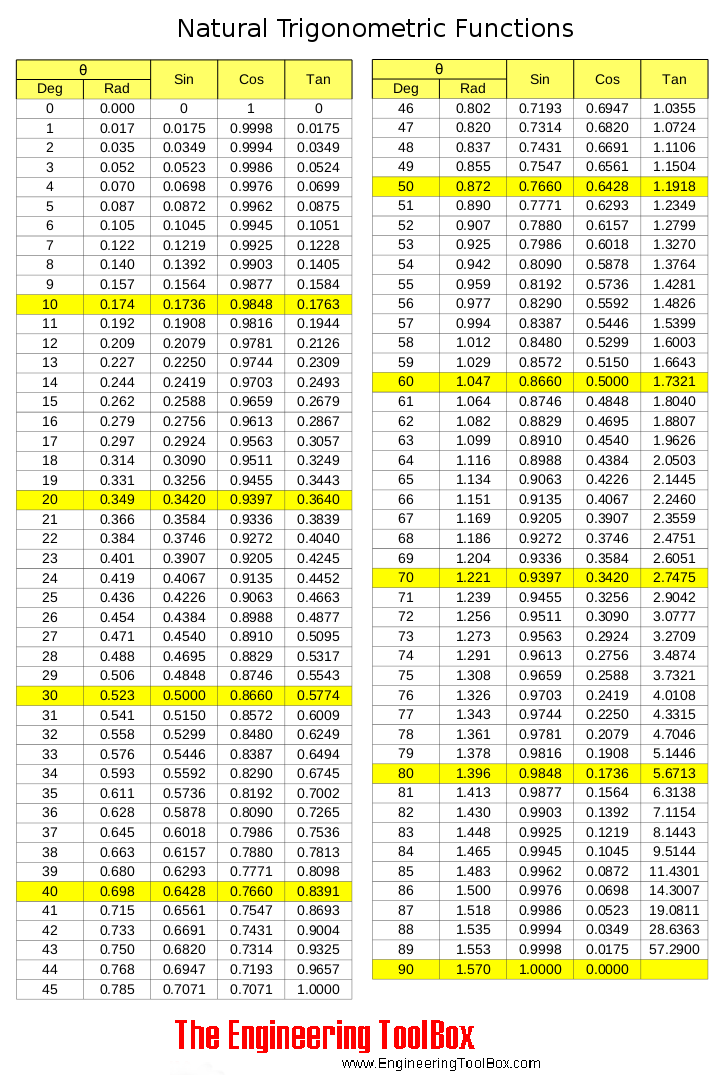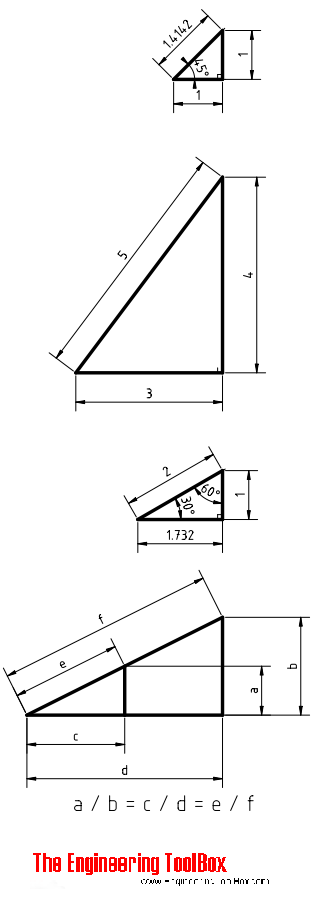Engineering ToolBox - Resources, Tools and Basic Information for Engineering and Design of Technical Applications!

# Trigonometric Functions

## Sine, cosine and tangent - the natural trigonometric functions.Natural trigonometric functions are expressed as

sin(θd) = a / c

= 1 / csc(θd)

= cos(π / 2 - θr)                       (1)

where

θd = angle in degrees

a

c

cos(θd) = b / c

= 1 / sec(θd)

= sin(π / 2 - θr)                       (2)

b

c

tan(θd) = a / b

= 1 / cot(θd)

= sin(θd) / cos(θd)

= cot(π / 2 - θr)                         (3)

a

b

cot(θd) = 1 / tan(θd)

= cos(θd) / sin(θd)

= tan(π / 2 - θr)                          (4)

θ (degrees)

Trigonometric functions ranging 0 to 90 degrees are tabulated below:### Inverse functions

arcsin (a) = sin-1 (a)                            (1a)

arccos (a) = cos-1 (a)                           (2a)

arctan (a) = tan-1 (a)                           (3a)

sin (a ± b) = sin (a) cos (b) ± cos (a) sin (b)                             (5)

cos (a ± b) = cos (a) cos (b) ± sin (a) sin (b)                            (5b)

tan (a ± b) = (tan(a) ± tan(b)) / (1 ± tan(a) tan(b))                         (5c)

### Sum and Difference Formula

sin (a) + sin (b) = 2 sin ((a + b)/2) cos ((a + b)/2)                          (6)

sin (a) - sin (b) = 2 cos ((a + b)/2) sin ((a - b)/2)                          (6b)

cos (a) + cos (b) = 2 cos ((a + b)/2) cos ((a - b)/2)                          (6c)

cos (a) - cos (b) = - 2 sin ((a + b)/2) sin ((a - b)/2)                         (6d)

tan (a) + tan (b) = sin (a + b) / (cos (a) cos (b))                       (6e)

tan (a) - tan (b) = sin (a - b) / (cos (a) cos (b))                    (6f)

### Product Formula

2 sin (a) cos (b) = sin (a - b) + sin (a + b)                       (7)

2 sin (a) sin (b) = cos (a - b) - cos (a - b)                      (7b)

2 cos (a) cos (b) = cos (a - b) + cos (a + b)                      (7c)

### Multiple Angle and Powers Formula

sin (2 a) = 2 sin (a) cos (a)                        (8)

cos (2 a) = cos2 (a) - sin2 (a)                        (8b)

cos (2 a) = 2 cos2 (a) - 1                          (8c)

cos (2 a) = 1 - 2 sin2 (a)                        (8d)

tan (2 a) = 2 tan a / (1 - tan2 (a))                     (8e)

sin2 (a) + cos2 (a) = 1                         (8f)

sec2 (a) = tan2 (a) + 1                         (8g)

### Special Triangles### Trigonometric Values

sin(-θd) = - sin(θd)                 (9a)

where

θd = angle in degrees

sin(90° + θd) = cos(θd)           (9b)

sin(90° - θd) = cos(θd)           (9c)

sin(180° + θd) = - sin(θd)           (9d)

sin(180° - θd) = sin(θd)           (9e)

sin(270° + θd) = - cos(θd)           (9f)

sin(270° - θd) = - cos(θd)          (9g)

sin(360° + θd) = sin(θd)          (9h)

sin(360° - θd) = - sin(θd)          (9h)

cos(-θd) = cos(θd)                (10a)

cos(90° + θd) = - sin(θd)           (10b)

cos(90° - θd) = sin(θd)          (10c)

cos(180° + θd) = - cos(θd)          (10d)

cos(180° - θd) = - cos(θd)          (10e)

cos(270° + θd) = sin(θd)           (10f)

cos(270° - θd) = - sin(θd)          (10g)

cos(360° + θd) = cos(θd)           (10h)

cos(360° - θd) = cos(θd)           (10h)

tan(-θd) = - tan(θd)                (11a)

tan(90° + θd) = - cot(θd)           (11b)

tan(90° - θd) = cot(θd)           (11c)

tan(180° + θd) = tan(θd)           (11d)

tan(180° - θd) = - tan(θd)            (11e)

tan(270° + θd) = - cot(θd)           (11f)

tan(270° - θd) = cot(θd)           (11g)

tan(360° + θd) = tan(θd)            (11h)

tan(360° - θd) = - tan(θd)           (11h)

### Trigometric Functions of Common Angles

30°45°60°90°
Sin 0 1 / 2 √2 / 2 √3 / 2 1
Cos 1 √2 / 2 √2 / 2 1 / 2 0
Tan 0 √3 / 3 1 √3
Cot √3 1 √3 / 3 0
Sec 1 2 √3 / 3 √2 2
Cosec 2 √2 2 √3 / 3 1

## Related Topics

• ### Basics

The SI-system, unit converters, physical constants, drawing scales and more.
• ### Mathematics

Mathematical rules and laws - numbers, areas, volumes, exponents, trigonometric functions and more.

## Related Documents

• ### Algebraic Expressions

Principal algebraic expressions formulas.
• ### Angle Calculator - Carpenter's Square

Calculate angles with a straight board across carpenter's square.
• ### Angle Converter

Converting between angle units.
• ### Centroids of Plane Areas

The controid of square, rectangle, circle, semi-circle and right-angled triangle.
• ### Complex Numbers

Complex numbers are used in alternating current theory and mechanical vector analysis.
• ### Fractions

Law of fractions
• ### Geometric Shapes - Areas

Areas, diagonals and more - of geometric figures like rectangles, triangles, trapezoids ..
• ### Hyperbolic Functions

Exponential functions related to the hyperbola.
• ### Law of Cosines

One side of a triangle when the opposite angle and two sides are known.
• ### Law of Tangents

Triangles and law of tangents.
• ### Laws of Indices

Simplifying calculations by involving indices.
• ### Logarithms

The rules of logarithms - log10 and loge for numbers ranging 1 to 1000.
• ### Miter Saw - Calculate Sawing Angle

Calculate miter saw protractor angles for skirting and decorative mouldings work.
• ### Numbers - Square, Cube, Square Root and Cubic Root Calculator

Calculate square, cube, square root and cubic root. Values tabulated for numbers ranging 1 to 100.
• ### Numbers - Squares, Cubes and Roots

Numbers - squares, cubes, square roots and cube roots.
• ### Oblique Triangle

Calculate oblique triangles.
• ### Pythagorean Theorem

Verifying square corners.

Radian is the SI unit of angle. Convert between degrees and radians. Calculate angular velocity.
• ### Right Angled Triangle

Right angled triangle equations.
• ### Soil - Earth Weight and Composition

Typical weight and composition of soil.
• ### Squaring with Diagonal Measurements

A rectangle is square if the lengths of both diagonals are equal.
• ### Standard Differentials and Integrals

Equations for differentials and integrals.
• ### Taylor Series

Function as an infinite sum of terms.

Online vector calculator - add vectors with different magnitude and direction - like forces, velocities and more.

## Engineering ToolBox - SketchUp Extension - Online 3D modeling!

Add standard and customized parametric components - like flange beams, lumbers, piping, stairs and more - to your Sketchup model with the Engineering ToolBox - SketchUp Extension - enabled for use with older versions of the amazing SketchUp Make and the newer "up to date" SketchUp Pro . Add the Engineering ToolBox extension to your SketchUp Make/Pro from the Extension Warehouse !

We don't collect information from our users. More about

## Citation

• The Engineering ToolBox (2008). Trigonometric Functions. [online] Available at: https://www.engineeringtoolbox.com/natural-trigonometric-functions-d_1124.html [Accessed Day Month Year].

Modify the access date according your visit.

9.19.12